# Selina Solutions Concise Maths Class 7 Chapter 2: Rational Numbers Exercise 2D

Selina Solutions Concise Maths Class 7 Chapter 2 Rational Numbers Exercise 2D provides students with a clear idea about the method of solving rational numbers using multiplication, multiplicative inverse and division. Students are advised to refer to the PDF of solutions in order to help them grasp the shortcut methods and important formulas. The solutions are provided in a step wise manner as each step carries marks in the annual exam. It is a key source of reference material for the students to improve their academic performance. Students can download Selina Solutions Concise Maths Class 7 Chapter 2 Rational Numbers Exercise 2D PDF, from the links given here.

## Selina Solutions Concise Maths Class 7 Chapter 2: Rational Numbers Exercise 2D Download PDF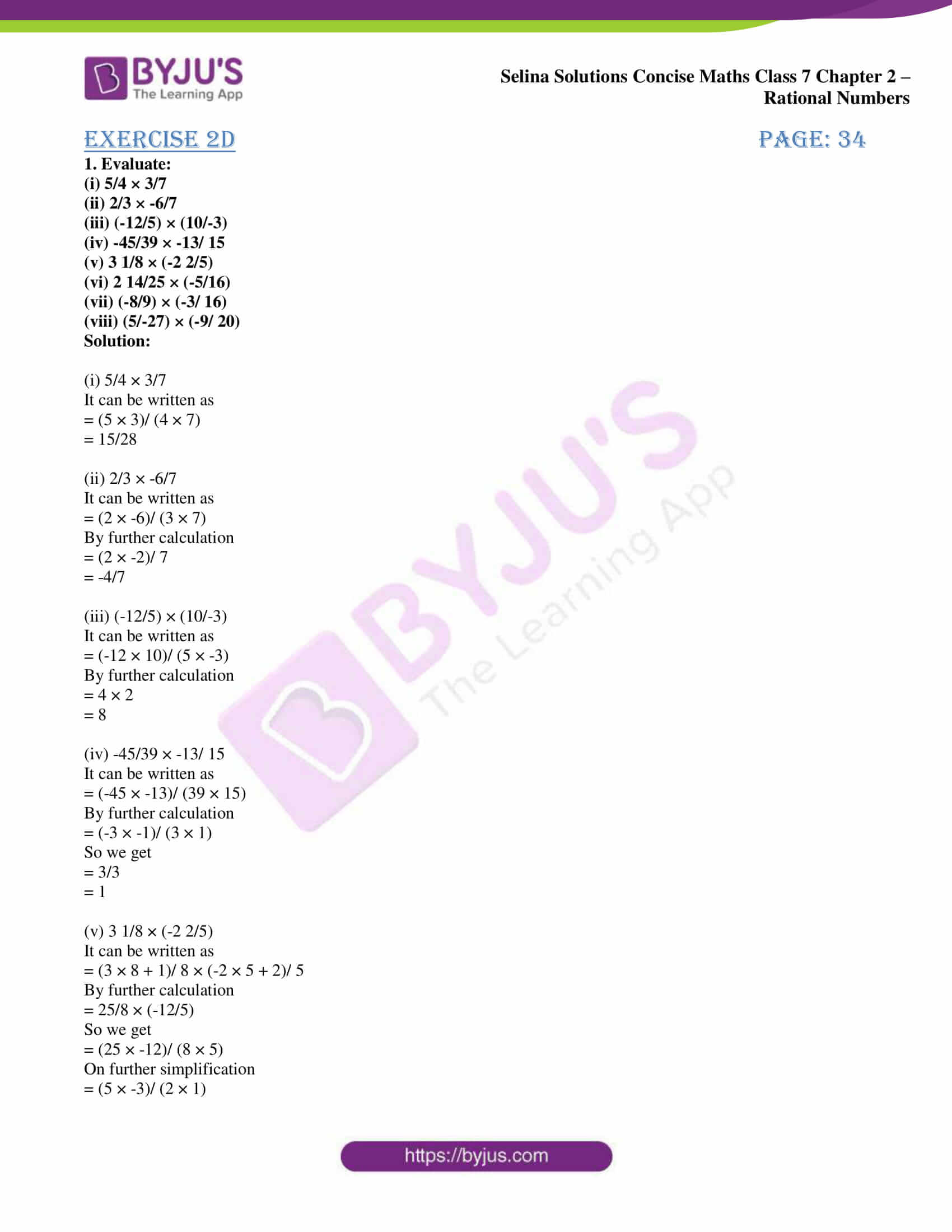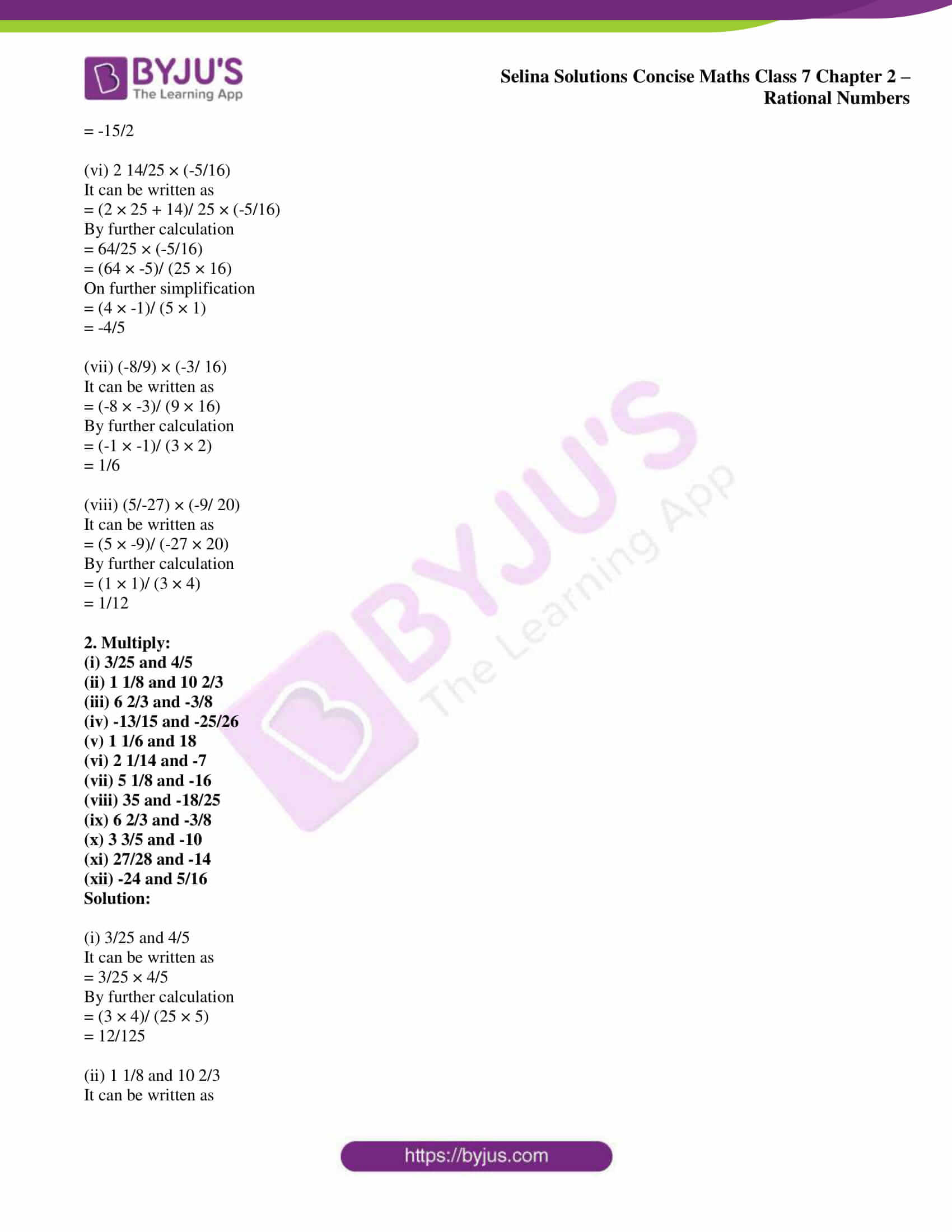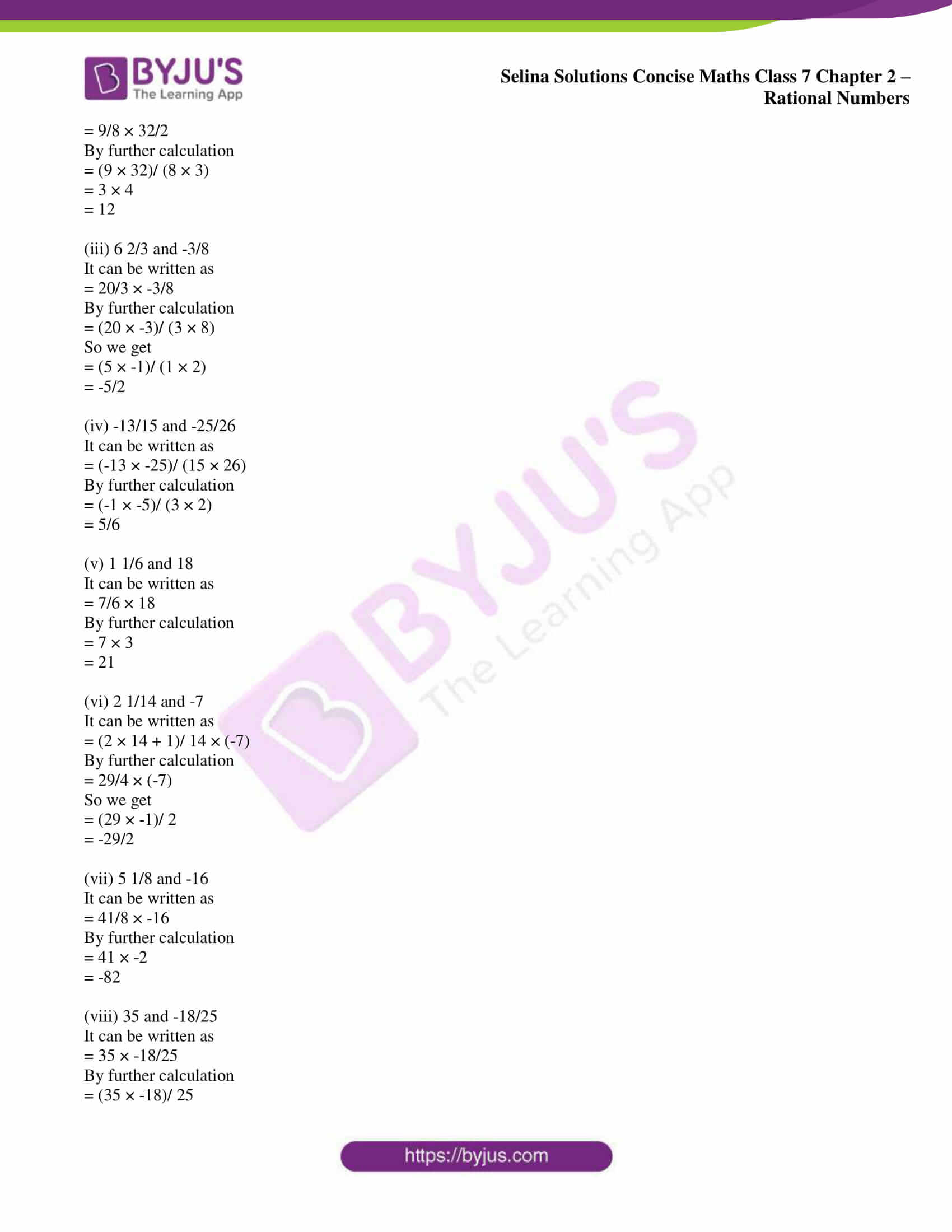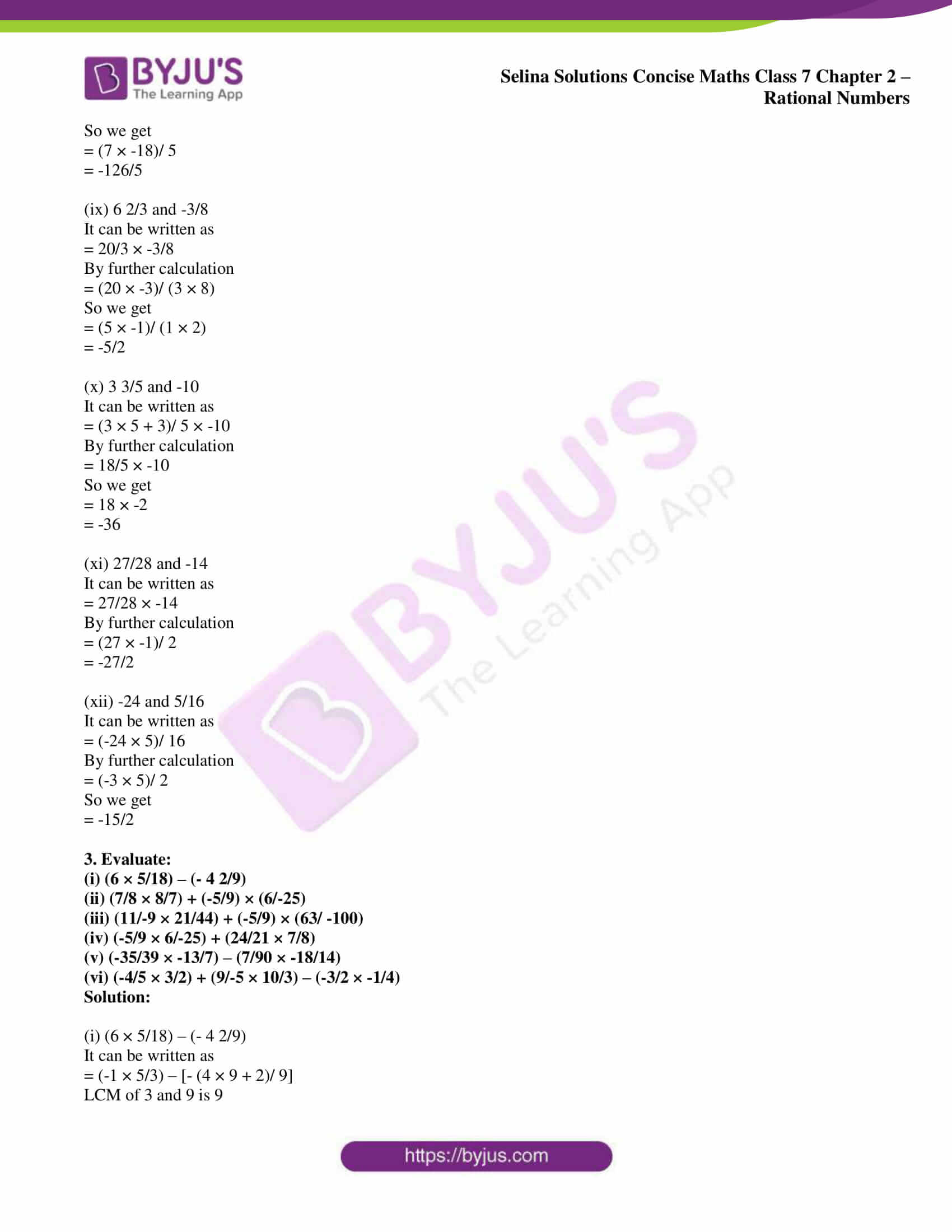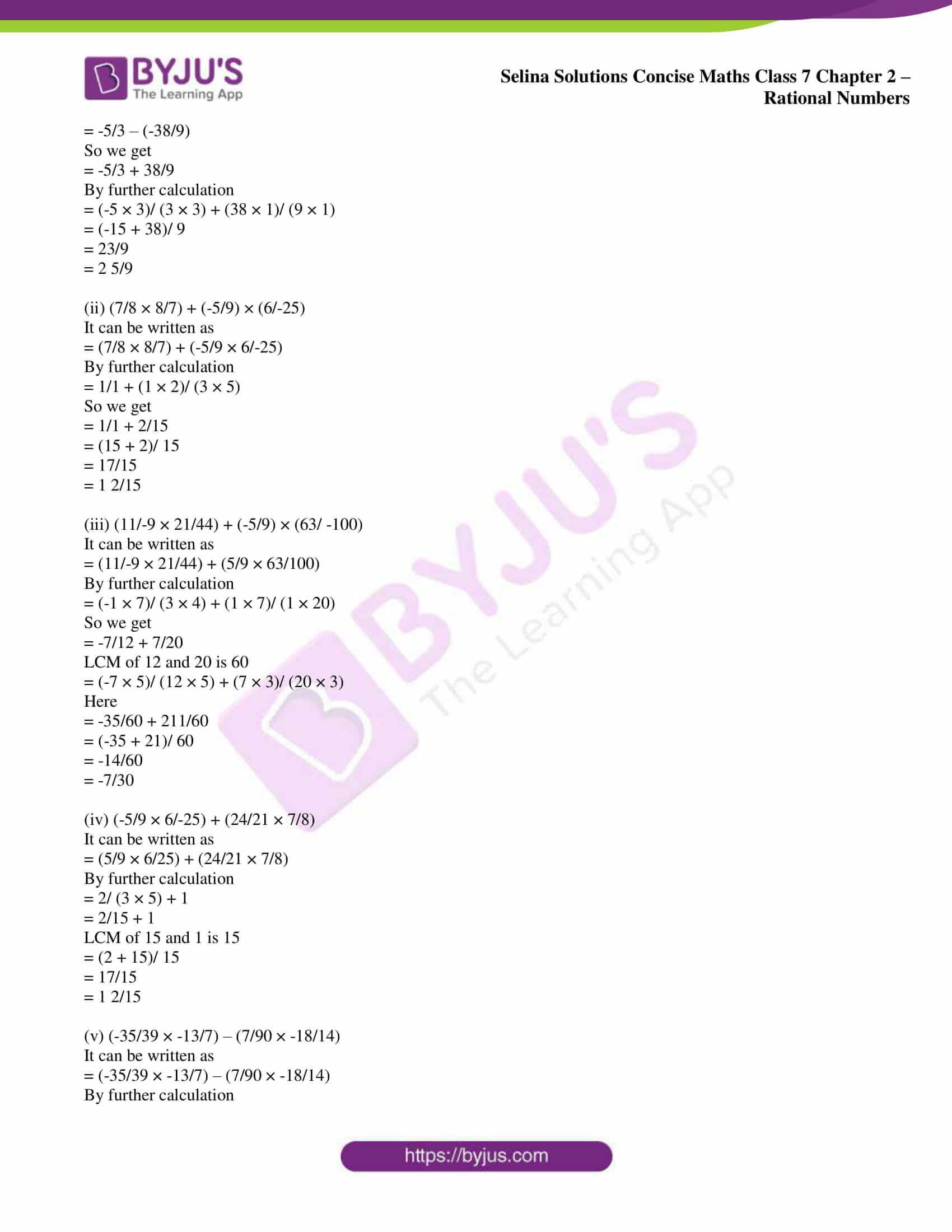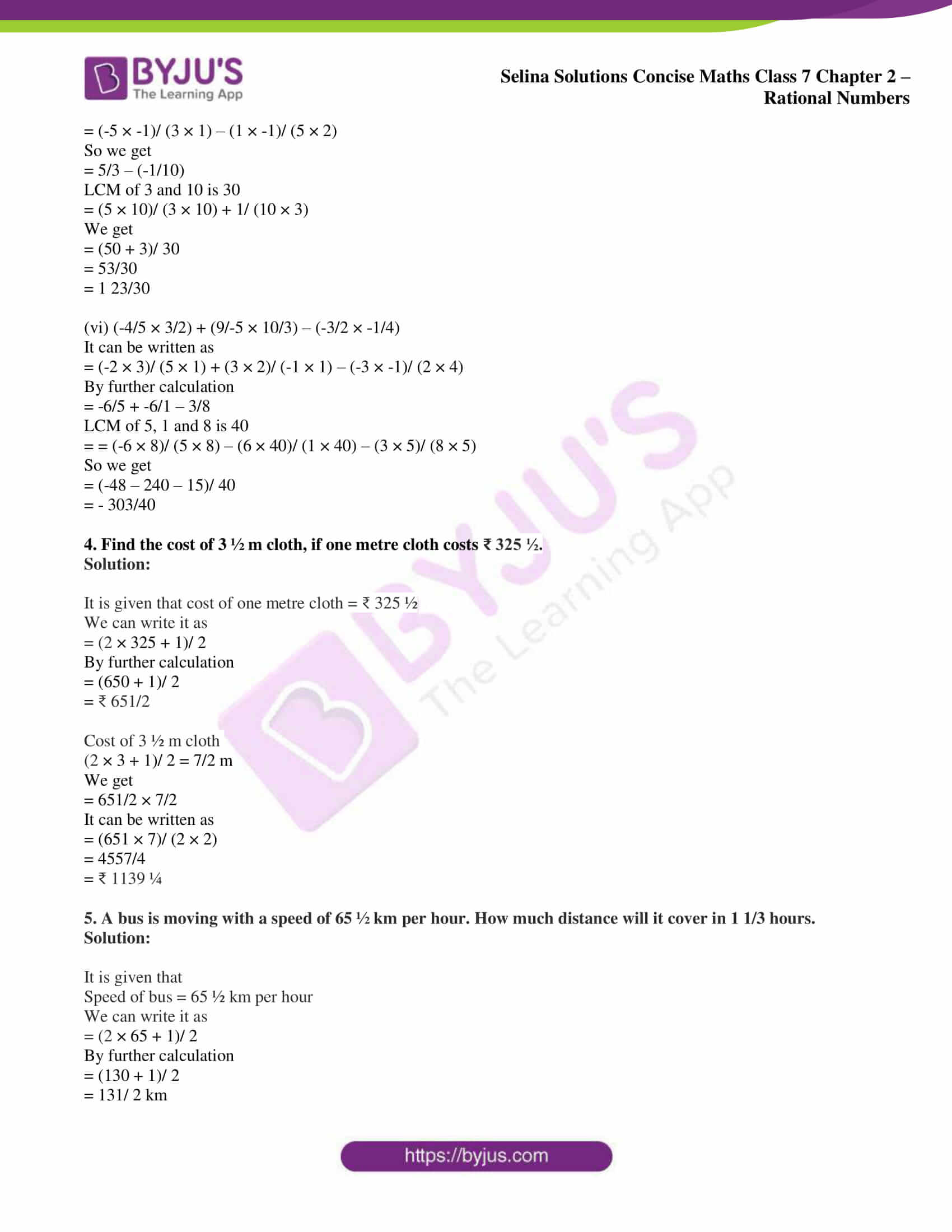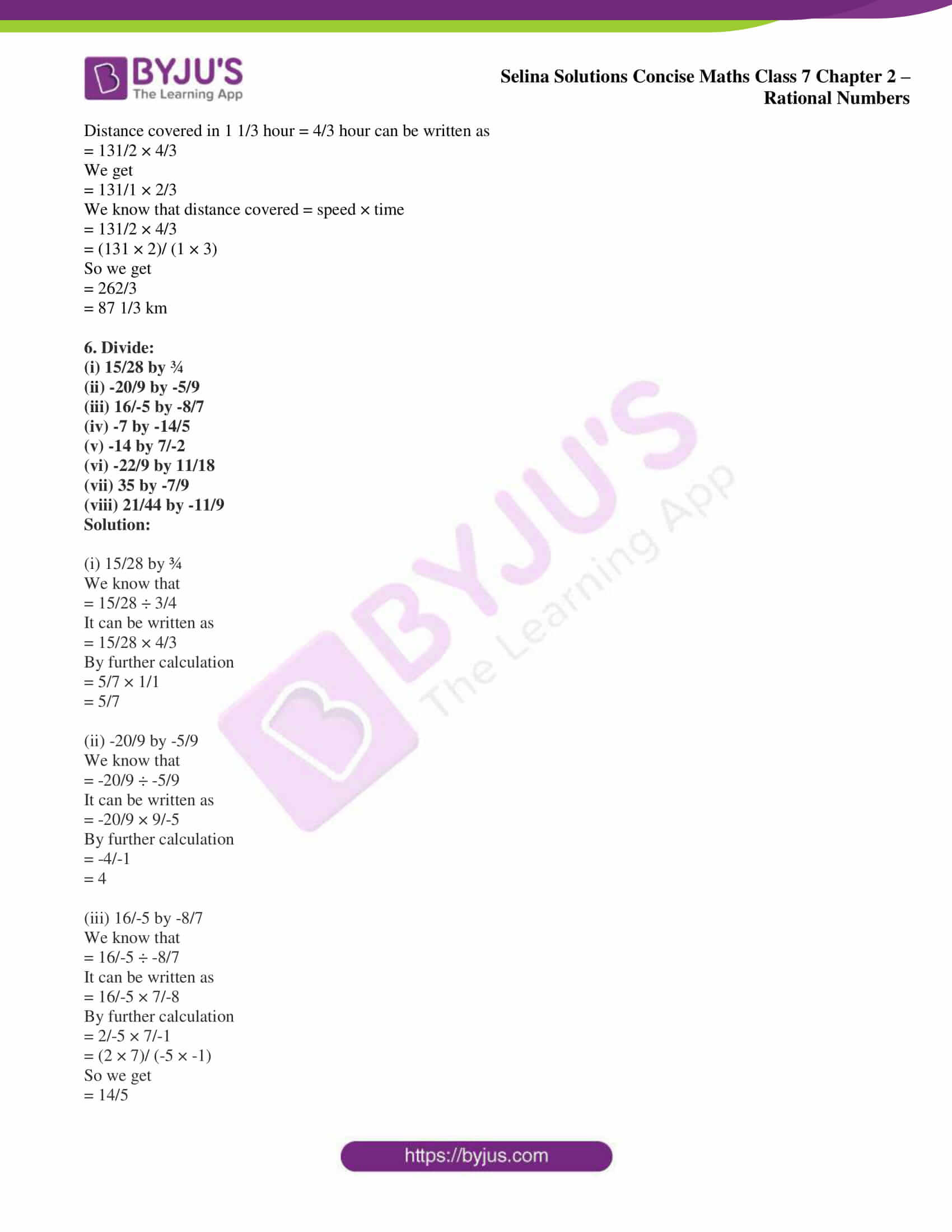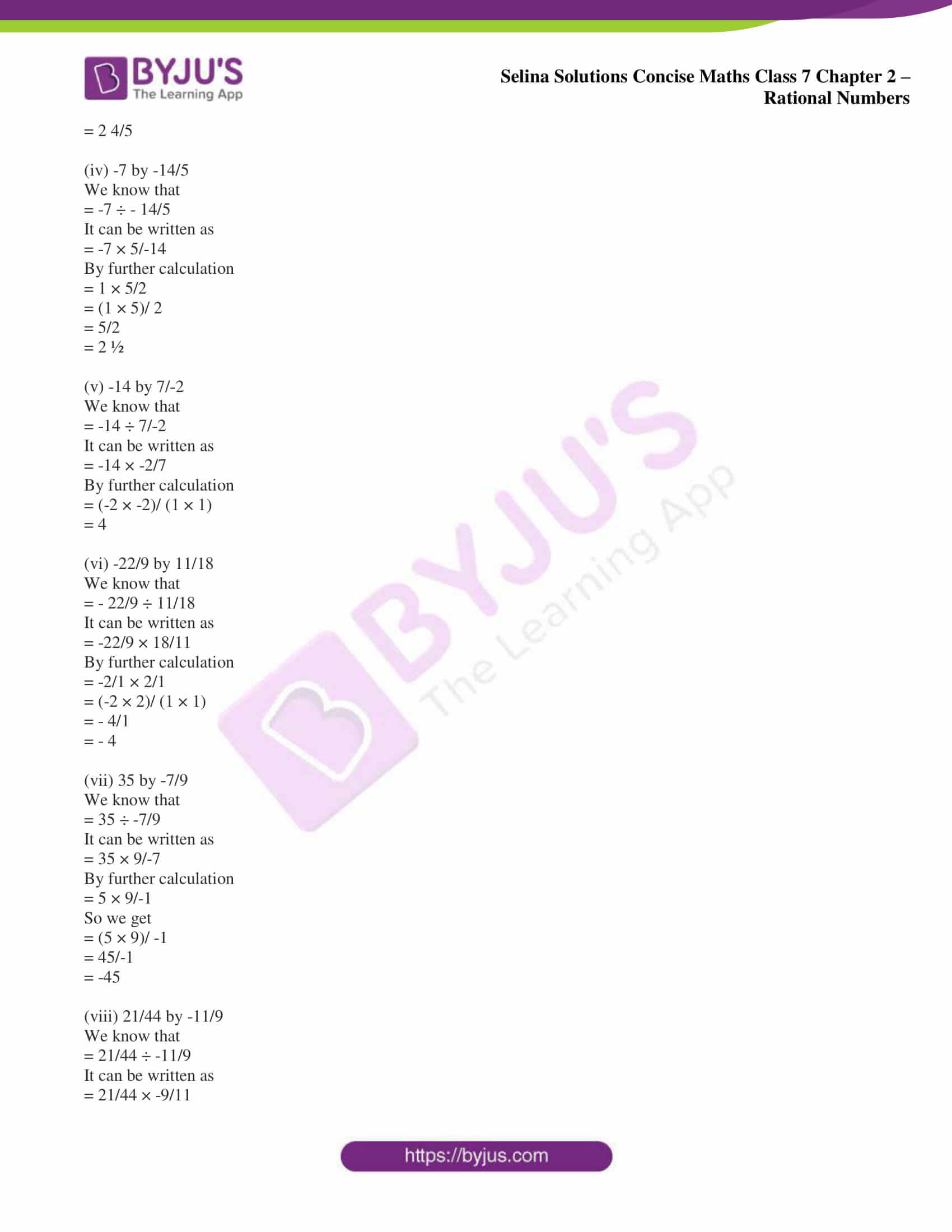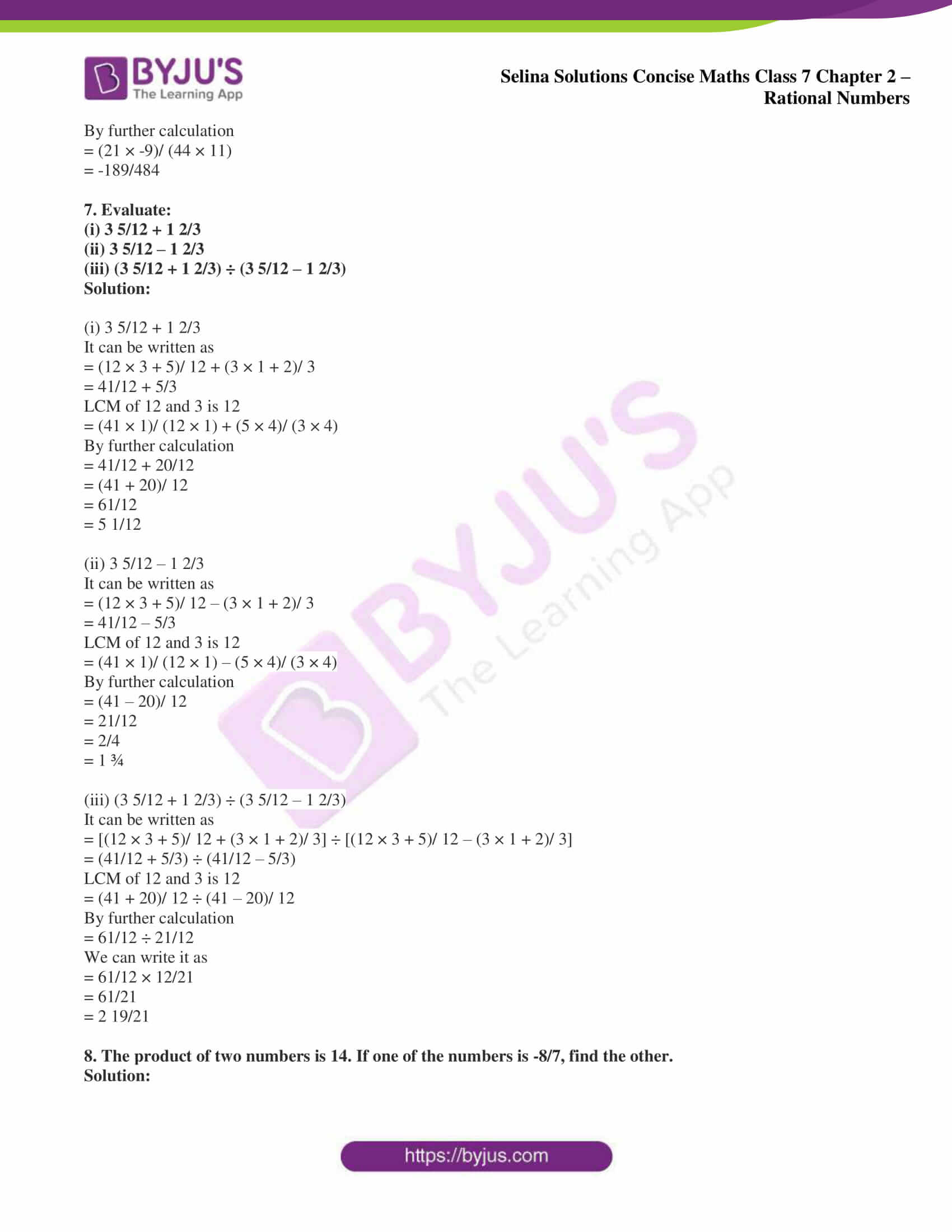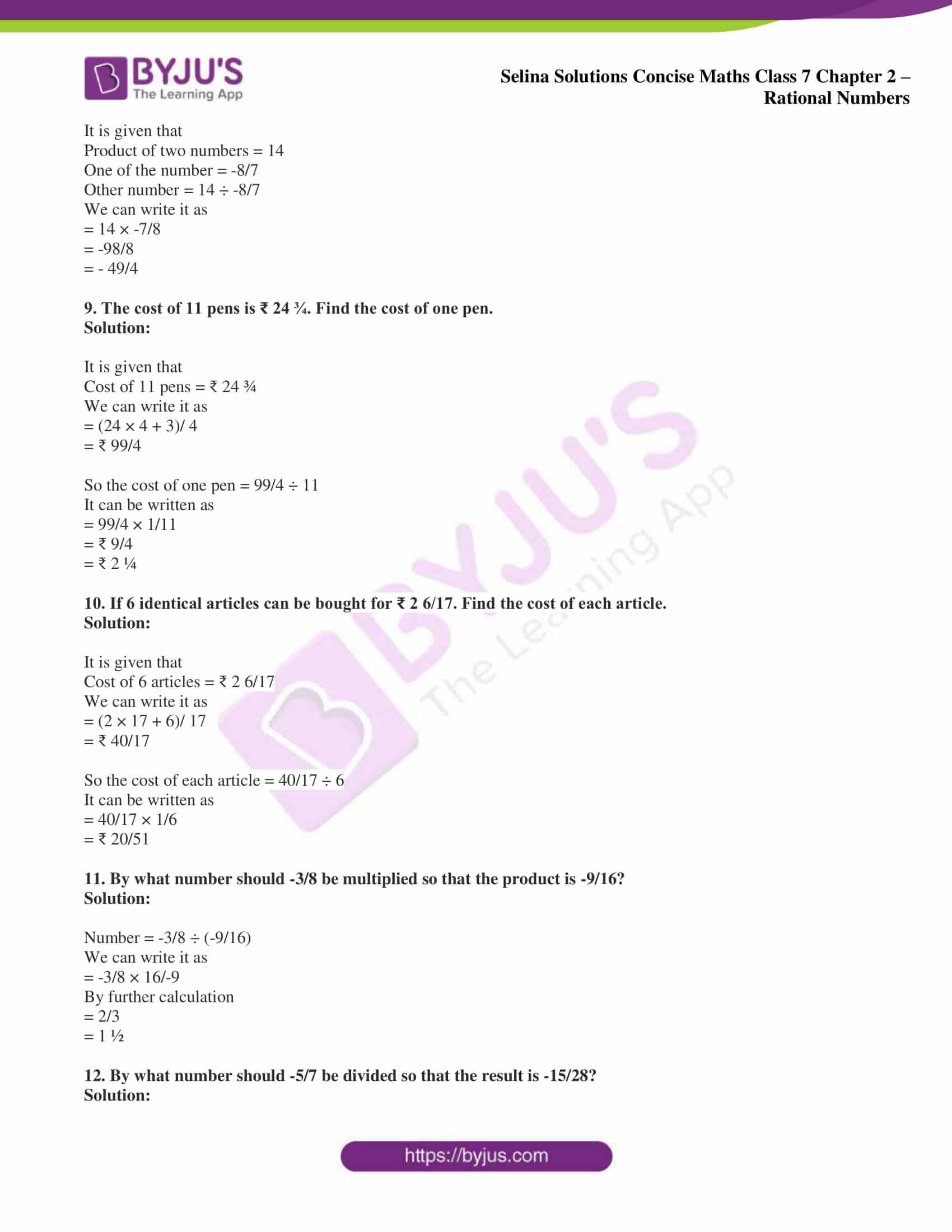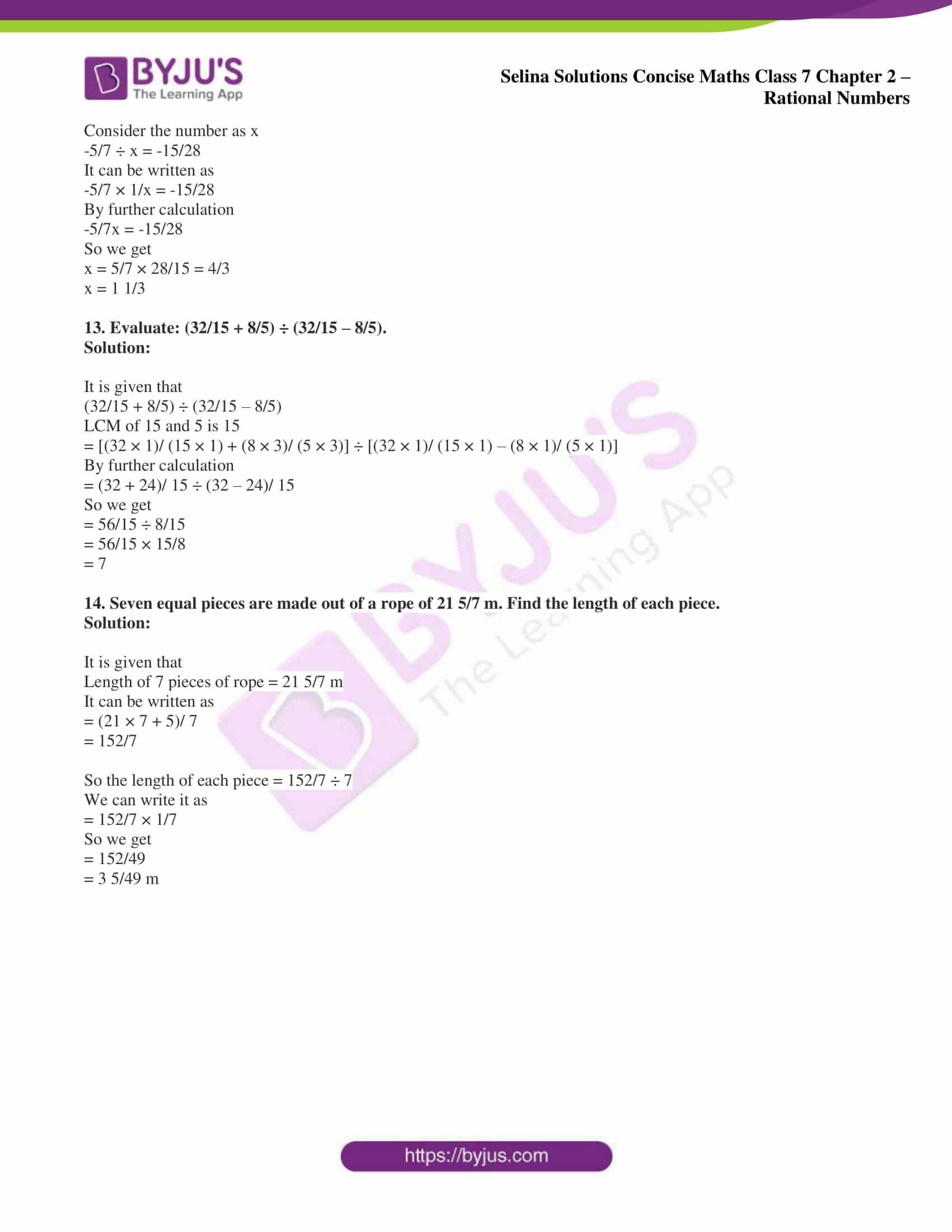### Access Selina Solutions Concise Maths Class 7 Chapter 2: Rational Numbers Exercise 2D

1. Evaluate:

(i) 5/4 × 3/7

(ii) 2/3 × -6/7

(iii) (-12/5) × (10/-3)

(iv) -45/39 × -13/ 15

(v) 3 1/8 × (-2 2/5)

(vi) 2 14/25 × (-5/16)

(vii) (-8/9) × (-3/ 16)

(viii) (5/-27) × (-9/ 20)

Solution:

(i) 5/4 × 3/7

It can be written as

= (5 × 3)/ (4 × 7)

= 15/28

(ii) 2/3 × -6/7

It can be written as

= (2 × -6)/ (3 × 7)

By further calculation

= (2 × -2)/ 7

= -4/7

(iii) (-12/5) × (10/-3)

It can be written as

= (-12 × 10)/ (5 × -3)

By further calculation

= 4 × 2

= 8

(iv) -45/39 × -13/ 15

It can be written as

= (-45 × -13)/ (39 × 15)

By further calculation

= (-3 × -1)/ (3 × 1)

So we get

= 3/3

= 1

(v) 3 1/8 × (-2 2/5)

It can be written as

= (3 × 8 + 1)/ 8 × (-2 × 5 + 2)/ 5

By further calculation

= 25/8 × (-12/5)

So we get

= (25 × -12)/ (8 × 5)

On further simplification

= (5 × -3)/ (2 × 1)

= -15/2

(vi) 2 14/25 × (-5/16)

It can be written as

= (2 × 25 + 14)/ 25 × (-5/16)

By further calculation

= 64/25 × (-5/16)

= (64 × -5)/ (25 × 16)

On further simplification

= (4 × -1)/ (5 × 1)

= -4/5

(vii) (-8/9) × (-3/ 16)

It can be written as

= (-8 × -3)/ (9 × 16)

By further calculation

= (-1 × -1)/ (3 × 2)

= 1/6

(viii) (5/-27) × (-9/ 20)

It can be written as

= (5 × -9)/ (-27 × 20)

By further calculation

= (1 × 1)/ (3 × 4)

= 1/12

2. Multiply:

(i) 3/25 and 4/5

(ii) 1 1/8 and 10 2/3

(iii) 6 2/3 and -3/8

(iv) -13/15 and -25/26

(v) 1 1/6 and 18

(vi) 2 1/14 and -7

(vii) 5 1/8 and -16

(viii) 35 and -18/25

(ix) 6 2/3 and -3/8

(x) 3 3/5 and -10

(xi) 27/28 and -14

(xii) -24 and 5/16

Solution:

(i) 3/25 and 4/5

It can be written as

= 3/25 × 4/5

By further calculation

= (3 × 4)/ (25 × 5)

= 12/125

(ii) 1 1/8 and 10 2/3

It can be written as

= 9/8 × 32/2

By further calculation

= (9 × 32)/ (8 × 3)

= 3 × 4

= 12

(iii) 6 2/3 and -3/8

It can be written as

= 20/3 × -3/8

By further calculation

= (20 × -3)/ (3 × 8)

So we get

= (5 × -1)/ (1 × 2)

= -5/2

(iv) -13/15 and -25/26

It can be written as

= (-13 × -25)/ (15 × 26)

By further calculation

= (-1 × -5)/ (3 × 2)

= 5/6

(v) 1 1/6 and 18

It can be written as

= 7/6 × 18

By further calculation

= 7 × 3

= 21

(vi) 2 1/14 and -7

It can be written as

= (2 × 14 + 1)/ 14 × (-7)

By further calculation

= 29/4 × (-7)

So we get

= (29 × -1)/ 2

= -29/2

(vii) 5 1/8 and -16

It can be written as

= 41/8 × -16

By further calculation

= 41 × -2

= -82

(viii) 35 and -18/25

It can be written as

= 35 × -18/25

By further calculation

= (35 × -18)/ 25

So we get

= (7 × -18)/ 5

= -126/5

(ix) 6 2/3 and -3/8

It can be written as

= 20/3 × -3/8

By further calculation

= (20 × -3)/ (3 × 8)

So we get

= (5 × -1)/ (1 × 2)

= -5/2

(x) 3 3/5 and -10

It can be written as

= (3 × 5 + 3)/ 5 × -10

By further calculation

= 18/5 × -10

So we get

= 18 × -2

= -36

(xi) 27/28 and -14

It can be written as

= 27/28 × -14

By further calculation

= (27 × -1)/ 2

= -27/2

(xii) -24 and 5/16

It can be written as

= (-24 × 5)/ 16

By further calculation

= (-3 × 5)/ 2

So we get

= -15/2

3. Evaluate:

(i) (6 × 5/18) – (- 4 2/9)

(ii) (7/8 × 8/7) + (-5/9) × (6/-25)

(iii) (11/-9 × 21/44) + (-5/9) × (63/ -100)

(iv) (-5/9 × 6/-25) + (24/21 × 7/8)
(v) (-35/39 × -13/7) – (7/90 × -18/14)

(vi) (-4/5 × 3/2) + (9/-5 × 10/3) – (-3/2 × -1/4)

Solution:

(i) (6 × 5/18) – (- 4 2/9)

It can be written as

= (-1 × 5/3) – [- (4 × 9 + 2)/ 9]

LCM of 3 and 9 is 9

= -5/3 – (-38/9)

So we get

= -5/3 + 38/9

By further calculation

= (-5 × 3)/ (3 × 3) + (38 × 1)/ (9 × 1)

= (-15 + 38)/ 9

= 23/9

= 2 5/9

(ii) (7/8 × 8/7) + (-5/9) × (6/-25)

It can be written as

= (7/8 × 8/7) + (-5/9 × 6/-25)

By further calculation

= 1/1 + (1 × 2)/ (3 × 5)

So we get

= 1/1 + 2/15

= (15 + 2)/ 15

= 17/15

= 1 2/15

(iii) (11/-9 × 21/44) + (-5/9) × (63/ -100)

It can be written as

= (11/-9 × 21/44) + (5/9 × 63/100)

By further calculation

= (-1 × 7)/ (3 × 4) + (1 × 7)/ (1 × 20)

So we get

= -7/12 + 7/20

LCM of 12 and 20 is 60

= (-7 × 5)/ (12 × 5) + (7 × 3)/ (20 × 3)

Here

= -35/60 + 211/60

= (-35 + 21)/ 60

= -14/60

= -7/30

(iv) (-5/9 × 6/-25) + (24/21 × 7/8)

It can be written as

= (5/9 × 6/25) + (24/21 × 7/8)

By further calculation

= 2/ (3 × 5) + 1

= 2/15 + 1

LCM of 15 and 1 is 15

= (2 + 15)/ 15

= 17/15

= 1 2/15

(v) (-35/39 × -13/7) – (7/90 × -18/14)

It can be written as

= (-35/39 × -13/7) – (7/90 × -18/14)

By further calculation

= (-5 × -1)/ (3 × 1) – (1 × -1)/ (5 × 2)

So we get

= 5/3 – (-1/10)

LCM of 3 and 10 is 30

= (5 × 10)/ (3 × 10) + 1/ (10 × 3)

We get

= (50 + 3)/ 30

= 53/30

= 1 23/30

(vi) (-4/5 × 3/2) + (9/-5 × 10/3) – (-3/2 × -1/4)

It can be written as

= (-2 × 3)/ (5 × 1) + (3 × 2)/ (-1 × 1) – (-3 × -1)/ (2 × 4)

By further calculation

= -6/5 + -6/1 – 3/8

LCM of 5, 1 and 8 is 40

= = (-6 × 8)/ (5 × 8) – (6 × 40)/ (1 × 40) – (3 × 5)/ (8 × 5)

So we get

= (-48 – 240 – 15)/ 40

= – 303/40

4. Find the cost of 3 ½ m cloth, if one metre cloth costs ₹ 325 ½.

Solution:

It is given that cost of one metre cloth = ₹ 325 ½

We can write it as

= (2 × 325 + 1)/ 2

By further calculation

= (650 + 1)/ 2

= ₹ 651/2

Cost of 3 ½ m cloth

(2 × 3 + 1)/ 2 = 7/2 m

We get

= 651/2 × 7/2

It can be written as

= (651 × 7)/ (2 × 2)

= 4557/4

= ₹ 1139 ¼

5. A bus is moving with a speed of 65 ½ km per hour. How much distance will it cover in 1 1/3 hours.

Solution:

It is given that

Speed of bus = 65 ½ km per hour

We can write it as

= (2 × 65 + 1)/ 2

By further calculation

= (130 + 1)/ 2

= 131/ 2 km

Distance covered in 1 1/3 hour = 4/3 hour can be written as

= 131/2 × 4/3

We get

= 131/1 × 2/3

We know that distance covered = speed × time

= 131/2 × 4/3

= (131 × 2)/ (1 × 3)

So we get

= 262/3

= 87 1/3 km

6. Divide:

(i) 15/28 by ¾

(ii) -20/9 by -5/9

(iii) 16/-5 by -8/7

(iv) -7 by -14/5

(v) -14 by 7/-2

(vi) -22/9 by 11/18

(vii) 35 by -7/9

(viii) 21/44 by -11/9

Solution:

(i) 15/28 by ¾

We know that

= 15/28 ÷ 3/4

It can be written as

= 15/28 × 4/3

By further calculation

= 5/7 × 1/1

= 5/7

(ii) -20/9 by -5/9

We know that

= -20/9 ÷ -5/9

It can be written as

= -20/9 × 9/-5

By further calculation

= -4/-1

= 4

(iii) 16/-5 by -8/7

We know that

= 16/-5 ÷ -8/7

It can be written as

= 16/-5 × 7/-8

By further calculation

= 2/-5 × 7/-1

= (2 × 7)/ (-5 × -1)

So we get

= 14/5

= 2 4/5

(iv) -7 by -14/5

We know that

= -7 ÷ – 14/5

It can be written as

= -7 × 5/-14

By further calculation

= 1 × 5/2

= (1 × 5)/ 2

= 5/2

= 2 ½

(v) -14 by 7/-2

We know that

= -14 ÷ 7/-2

It can be written as

= -14 × -2/7

By further calculation

= (-2 × -2)/ (1 × 1)

= 4

(vi) -22/9 by 11/18

We know that

= – 22/9 ÷ 11/18

It can be written as

= -22/9 × 18/11

By further calculation

= -2/1 × 2/1

= (-2 × 2)/ (1 × 1)

= – 4/1

= – 4

(vii) 35 by -7/9

We know that

= 35 ÷ -7/9

It can be written as

= 35 × 9/-7

By further calculation

= 5 × 9/-1

So we get

= (5 × 9)/ -1

= 45/-1

= -45

(viii) 21/44 by -11/9

We know that

= 21/44 ÷ -11/9

It can be written as

= 21/44 × -9/11

By further calculation

= (21 × -9)/ (44 × 11)

= -189/484

7. Evaluate:

(i) 3 5/12 + 1 2/3

(ii) 3 5/12 – 1 2/3

(iii) (3 5/12 + 1 2/3) ÷ (3 5/12 – 1 2/3)

Solution:

(i) 3 5/12 + 1 2/3

It can be written as

= (12 × 3 + 5)/ 12 + (3 × 1 + 2)/ 3

= 41/12 + 5/3

LCM of 12 and 3 is 12

= (41 × 1)/ (12 × 1) + (5 × 4)/ (3 × 4)

By further calculation

= 41/12 + 20/12

= (41 + 20)/ 12

= 61/12

= 5 1/12

(ii) 3 5/12 – 1 2/3

It can be written as

= (12 × 3 + 5)/ 12 – (3 × 1 + 2)/ 3

= 41/12 – 5/3

LCM of 12 and 3 is 12

= (41 × 1)/ (12 × 1) – (5 × 4)/ (3 × 4)

By further calculation

= (41 – 20)/ 12

= 21/12

= 2/4

= 1 ¾

(iii) (3 5/12 + 1 2/3) ÷ (3 5/12 – 1 2/3)

It can be written as

= [(12 × 3 + 5)/ 12 + (3 × 1 + 2)/ 3] ÷ [(12 × 3 + 5)/ 12 – (3 × 1 + 2)/ 3]

= (41/12 + 5/3) ÷ (41/12 – 5/3)

LCM of 12 and 3 is 12

= (41 + 20)/ 12 ÷ (41 – 20)/ 12

By further calculation

= 61/12 ÷ 21/12

We can write it as

= 61/12 × 12/21

= 61/21

= 2 19/21

8. The product of two numbers is 14. If one of the numbers is -8/7, find the other.

Solution:

It is given that

Product of two numbers = 14

One of the number = -8/7

Other number = 14 ÷ -8/7

We can write it as

= 14 × -7/8

= -98/8

= – 49/4

9. The cost of 11 pens is ₹ 24 ¾. Find the cost of one pen.

Solution:

It is given that

Cost of 11 pens = ₹ 24 ¾

We can write it as

= (24 × 4 + 3)/ 4

= ₹ 99/4

So the cost of one pen = 99/4 ÷ 11

It can be written as

= 99/4 × 1/11

= ₹ 9/4

= ₹ 2 ¼

10. If 6 identical articles can be bought for ₹ 2 6/17. Find the cost of each article.

Solution:

It is given that

Cost of 6 articles = ₹ 2 6/17

We can write it as

= (2 × 17 + 6)/ 17

= ₹ 40/17

So the cost of each article = 40/17 ÷ 6

It can be written as

= 40/17 × 1/6

= ₹ 20/51

11. By what number should -3/8 be multiplied so that the product is -9/16?

Solution:

Number = -3/8 ÷ (-9/16)

We can write it as

= -3/8 × 16/-9

By further calculation

= 2/3

= 1 ½

12. By what number should -5/7 be divided so that the result is -15/28?

Solution:

Consider the number as x

-5/7 ÷ x = -15/28

It can be written as

-5/7 × 1/x = -15/28

By further calculation

-5/7x = -15/28

So we get

x = 5/7 × 28/15 = 4/3

x = 1 1/3

13. Evaluate: (32/15 + 8/5) ÷ (32/15 – 8/5).

Solution:

It is given that

(32/15 + 8/5) ÷ (32/15 – 8/5)

LCM of 15 and 5 is 15

= [(32 × 1)/ (15 × 1) + (8 × 3)/ (5 × 3)] ÷ [(32 × 1)/ (15 × 1) – (8 × 1)/ (5 × 1)]

By further calculation

= (32 + 24)/ 15 ÷ (32 – 24)/ 15

So we get

= 56/15 ÷ 8/15

= 56/15 × 15/8

= 7

14. Seven equal pieces are made out of a rope of 21 5/7 m. Find the length of each piece.

Solution:

It is given that

Length of 7 pieces of rope = 21 5/7 m

It can be written as

= (21 × 7 + 5)/ 7

= 152/7

So the length of each piece = 152/7 ÷ 7

We can write it as

= 152/7 × 1/7

So we get

= 152/49

= 3 5/49 m